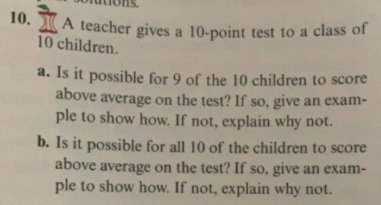# 10. A teacher gives a 10-point test to a class of10 children.a. Is it possible for 9 of the 10 children to scoreabove average on the test? If so, give an exam-ple to show how. If not, explain why not.b. Is it possible for all 10 of the children to scoreabove average on the test? If so, give an exam-ple to show how. If not, explain why not.

Question

15.3 #10help_outlineImage Transcriptionclose10. A teacher gives a 10-point test to a class of 10 children. a. Is it possible for 9 of the 10 children to score above average on the test? If so, give an exam- ple to show how. If not, explain why not. b. Is it possible for all 10 of the children to score above average on the test? If so, give an exam- ple to show how. If not, explain why not. fullscreen
check_circle

Step 1

The average is the sum of all data points and divided by total number of data points. the formula to calcuate the mean is shown below.

Step 2

Given a 10 point test to class of 10 students.
It is possible that 9 out of 10 children to score above average on test.
For example let 9 students scor...

### Want to see the full answer?

See Solution

#### Want to see this answer and more?

Solutions are written by subject experts who are available 24/7. Questions are typically answered within 1 hour.*

See Solution
*Response times may vary by subject and question.
Tagged in

### Measures